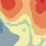# Understanding the equation of the mathematical models used to describe the semivariogram in a kriging analysis

242
1
3 weeks agoNew Contributor

Hi,

I'm using the kriging analysis tool in ArcMap (10.7.1) to be able to export the semivariogram graph values. For the analysis, I'm using ordinary kriging, Box-Cox transformation type with no trend, and the exponential mathematical model. I want to understand the equation behind this model and I'm confused about how to interpret the equation that appears below the semivariogram (attached below). Going through the documentation I found this web page:  There I saw the equation that is used for the exponential model, but when calculating the y-axis values using the model equation I do not obtain the same results that I exported from ArcMap.

I want to know as I'm trying to graph the values obtained but with the distance in kilometers (which I can convert from degrees to km) but to get the y-axis values for the model I want to compute them using the model equation.

I will appreciate any insight or help.

1 Solution

Accepted SolutionsbyEsri Regular Contributor

Hi Christina,

There is a lot going on with that equation, probably too much to summarize in a post.  The idea is that the red dots and blue crosses are estimated directly from the data, and the smooth blue semivariogram model is fit to this data (similar to fitting a regression line to a scatterplot).  The equation under the curve is the equation for the smooth blue line.

Some of the formulas used in Geostatistical Analyst are slightly different than used in 3D Analyst and Spatial Analyst (the help page you linked to).  You can find the exact formulas used in Geostatistical Analyst on page 263 of this document: https://dusk.geo.orst.edu/gis/geostat_analyst.pdf

However, I would highly suggest that you project your data to a projected coordinate system and perform kriging on the projected data.  In general, you cannot convert semivariograms calculated in one coordinate system to another coordinate system.  This problem is especially bad for data with latitude-longitude coordinates because there is no standard conversion between degrees and other linear units like kilometers.

Hope that helps,

-EricbyEsri Regular Contributor

Hi Christina,

There is a lot going on with that equation, probably too much to summarize in a post.  The idea is that the red dots and blue crosses are estimated directly from the data, and the smooth blue semivariogram model is fit to this data (similar to fitting a regression line to a scatterplot).  The equation under the curve is the equation for the smooth blue line.

Some of the formulas used in Geostatistical Analyst are slightly different than used in 3D Analyst and Spatial Analyst (the help page you linked to).  You can find the exact formulas used in Geostatistical Analyst on page 263 of this document: https://dusk.geo.orst.edu/gis/geostat_analyst.pdf

However, I would highly suggest that you project your data to a projected coordinate system and perform kriging on the projected data.  In general, you cannot convert semivariograms calculated in one coordinate system to another coordinate system.  This problem is especially bad for data with latitude-longitude coordinates because there is no standard conversion between degrees and other linear units like kilometers.

Hope that helps,

-Eric